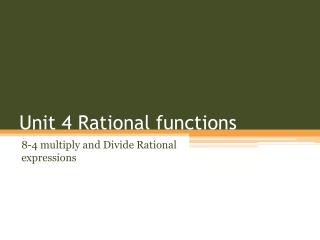# Unit 4 Rational functions - PowerPoint PPT PresentationDownload PresentationUnit 4 Rational functions

Unit 4 Rational functionsDownload Presentation## Unit 4 Rational functions

- - - - - - - - - - - - - - - - - - - - - - - - - - - E N D - - - - - - - - - - - - - - - - - - - - - - - - - - -
##### Presentation Transcript

1. Unit 4 Rational functions 8-4 multiply and Divide Rational expressions

2. Simplifying a Rational Expression Simplify each FACTOR

3. Multiply Rational expressions Simplify each

4. Dividing rational expressions • SAME , CHANGE , FLIP !!! Math at work

5. Simplify rational expressions

6. practice • Pg 531 #8-12 even 15-25 odd